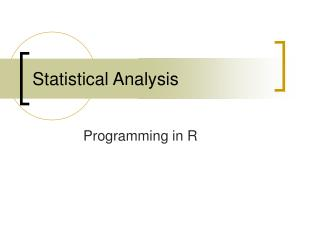Download PresentationStatistical AnalysisStatistical Analysis - PowerPoint PPT Presentation

Download PresentationStatistical Analysis
An Image/Link below is provided (as is) to download presentation

Download Policy: Content on the Website is provided to you AS IS for your information and personal use and may not be sold / licensed / shared on other websites without getting consent from its author. While downloading, if for some reason you are not able to download a presentation, the publisher may have deleted the file from their server.

- - - - - - - - - - - - - - - - - - - - - - - - - - - E N D - - - - - - - - - - - - - - - - - - - - - - - - - - -
Presentation Transcript

1. Statistical Analysis Programming in R

2. Vectors and assignment • Simplest data structure is the numeric vector: • Type at the command line: > x<-c(10.4, 5.6, 3.1, 6.4, 21.7) • Type x at the command line to see the result: > x  10.4 5.6 3.1 6.4 21.7 >

3. c() is a function • Function c() takes an arbitrary number of vector arguments and concatenates them. > y<-c(x, 0, x) > y  10.4 5.6 3.1 6.4 21.7 0.0 10.4 5.6 3.1 6.4 21.7

4. Vector arithmetic • +,x,*,/,^ • log, exp, sin, cos, tan, sqrt,… • max, min, range • length, sum, prod

5. Calculate mean in R: mean and variation > mean(x)  9.44 > > var(x)  53.853 >

6. Calculate mean in R: mean and variation • mean(x) can be written as: > sum(x)/length(x)  9.44 • var(x) can be written as: > sum((x-mean(x))^2)/(length(x)-1)  53.853

7. Two sample t-statistic twosam = function(y1,y2) { n1=length(y1); n2 =length(y2) yb1=mean(y1); yb2=mean(y2) s1=var(y1); s2=var(y2) s=((n1-1)*s1 + (n2-1)*s2)/(n1+n2-2) tst=(yb1-yb2)/sqrt(s2*(1/n1+1/n2)) tst } Copy and paste the above statements onto the command line in R

8. Should look like this: > twosam <- function(y1,y2) { + n1<-length(y1); n2 <-length(y2) + yb1=mean(y1); yb2=mean(y2) + s1=var(y1); s2=var(y2) + s=((n1-1)*s1 + (n2-1)*s2)/(n1+n2-2) + tst=(yb1-yb2)/sqrt(s2*(1/n1+1/n2)) + tst + }

9. Test your function by calling it: > tstat=twosam(x,x+1) > tstat  -0.2154592 >

10. Generating regular sequences • 1:30 is the same with c(1,2,3,…,29,30) • : operator has the highest priority within an expression. For example: > 2*1:5  2 4 6 8 10

11. factors > codons=c("GCA","GCC","GCG","GCU","UGC","UGU") > codons  "GCA" "GCC" "GCG" "GCU" "UGC" "UGU" > aminoacids=c("Ala","Ala","Ala","Ala","Cys","Cys") > aminoacids  "Ala" "Ala" "Ala" "Ala" "Cys" "Cys" > aaf=factor(aminoacids) > aaf  Ala Ala Ala Ala Cys Cys Levels: Ala Cys > ii=tapply(codons,aaf,print)  "GCA" "GCC" "GCG" "GCU"  "UGC" "UGU" >

12. arrays > x=array(1:20,dim=c(4,5)) > x [,1] [,2] [,3] [,4] [,5] [1,] 1 5 9 13 17 [2,] 2 6 10 14 18 [3,] 3 7 11 15 19 [4,] 4 8 12 16 20

13. arrays > x=array(0,dim=c(4,5)) > x [,1] [,2] [,3] [,4] [,5] [1,] 0 0 0 0 0 [2,] 0 0 0 0 0 [3,] 0 0 0 0 0 [4,] 0 0 0 0 0 >

14. Indexing arrays > i=array(c(1:3,3:1),dim=c(3,2)) > i [,1] [,2] [1,] 1 3 [2,] 2 2 [3,] 3 1 > x=array(1:20,dim=c(4,5)) > x [,1] [,2] [,3] [,4] [,5] [1,] 1 5 9 13 17 [2,] 2 6 10 14 18 [3,] 3 7 11 15 19 [4,] 4 8 12 16 20 > x[i]  9 6 3 > x[i]=0 > x [,1] [,2] [,3] [,4] [,5] [1,] 1 5 0 13 17 [2,] 2 0 10 14 18 [3,] 0 7 11 15 19 [4,] 4 8 12 16 20